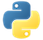# MLTransform for data processingPydoc

Use `MLTransform` to apply common machine learning (ML) processing tasks on keyed data. Apache Beam provides ML data processing transformations that you can use with `MLTransform`. For the full list of available data processing transformations, see the tft.py file in GitHub.

To define a data processing transformation by using `MLTransform`, create instances of data processing transforms with `columns` as input parameters. The data in the specified `columns` is transformed and outputted to the `beam.Row` object.

The following example demonstrates how to use `MLTransform` to normalize your data between 0 and 1 by using the minimum and maximum values from your entire dataset. `MLTransform` uses the `ScaleTo01` transformation.

``````scale_to_z_score_transform = ScaleToZScore(columns=['x', 'y'])
with beam.Pipeline() as p:
(data | MLTransform(write_artifact_location=artifact_location).with_transform(scale_to_z_score_transform))
``````

In this example, `MLTransform` receives a value for `write_artifact_location`. `MLTransform` then uses this location value to write artifacts generated by the transform. To pass the data processing transform, you can use either the `with_transform` method of `MLTransform` or a list.

``````MLTransform(transforms=transforms, write_artifact_location=write_artifact_location)
``````

The transforms passed to `MLTransform` are applied sequentially on the dataset. `MLTransform` expects a dictionary and returns a transformed row object with NumPy arrays.

## Examples

The following examples demonstrate how to to create pipelines that use `MLTransform` to preprocess data.

`MLTransform` can do a full pass on the dataset, which is useful when you need to transform a single element only after analyzing the entire dataset. The first two examples require a full pass over the dataset to complete the data transformation.

• For the `ComputeAndApplyVocabulary` transform, the transform needs access to all of the unique words in the dataset.
• For the `ScaleTo01` transform, the transform needs to know the minimum and maximum values in the dataset.

### Example 1

This example creates a pipeline that uses `MLTransform` to scale data between 0 and 1. The example takes a list of integers and converts them into the range of 0 to 1 using the transform `ScaleTo01`.``````import apache_beam as beam
from apache_beam.ml.transforms.base import MLTransform
from apache_beam.ml.transforms.tft import ScaleTo01
import tempfile

data = [
{
'x': [1, 5, 3]
},
{
'x': [4, 2, 8]
},
]

artifact_location = tempfile.mkdtemp()
scale_to_0_1_fn = ScaleTo01(columns=['x'])

with beam.Pipeline() as p:
transformed_data = (
p
| beam.Create(data)
| MLTransform(write_artifact_location=artifact_location).with_transform(
scale_to_0_1_fn)
| beam.Map(print))``````

Output:``````Row(x=array([0.       , 0.5714286, 0.2857143], dtype=float32), x_max=array([8.], dtype=float32), x_min=array([1.], dtype=float32))
Row(x=array([0.42857143, 0.14285715, 1.        ], dtype=float32), x_max=array([8.], dtype=float32), x_min=array([1.], dtype=float32))``````

### Example 2

This example creates a pipeline that use `MLTransform` to compute vocabulary on the entire dataset and assign indices to each unique vocabulary item. It takes a list of strings, computes vocabulary over the entire dataset, and then applies a unique index to each vocabulary item.``````import apache_beam as beam
from apache_beam.ml.transforms.base import MLTransform
from apache_beam.ml.transforms.tft import ComputeAndApplyVocabulary
import tempfile

artifact_location = tempfile.mkdtemp()
data = [
{
'x': ['I', 'love', 'Beam']
},
{
'x': ['Beam', 'is', 'awesome']
},
]
compute_and_apply_vocabulary_fn = ComputeAndApplyVocabulary(columns=['x'])
with beam.Pipeline() as p:
transformed_data = (
p
| beam.Create(data)
| MLTransform(write_artifact_location=artifact_location).with_transform(
compute_and_apply_vocabulary_fn)
| beam.Map(print))``````

Output:``````Row(x=array([4, 1, 0]))
Row(x=array([0, 2, 3]))``````

### Example 3

This example creates a pipeline that uses `MLTransform` to compute vocabulary on the entire dataset and assign indices to each unique vocabulary item. This pipeline takes a single element as input instead of a list of elements.``````import apache_beam as beam
from apache_beam.ml.transforms.base import MLTransform
from apache_beam.ml.transforms.tft import ComputeAndApplyVocabulary
import tempfile
data = [
{
'x': 'I'
},
{
'x': 'love'
},
{
'x': 'Beam'
},
{
'x': 'Beam'
},
{
'x': 'is'
},
{
'x': 'awesome'
},
]
artifact_location = tempfile.mkdtemp()
compute_and_apply_vocabulary_fn = ComputeAndApplyVocabulary(columns=['x'])
with beam.Pipeline() as p:
transformed_data = (
p
| beam.Create(data)
| MLTransform(write_artifact_location=artifact_location).with_transform(
compute_and_apply_vocabulary_fn)
| beam.Map(print))``````

Output:``````Row(x=array())
Row(x=array())
Row(x=array())
Row(x=array())
Row(x=array())``````